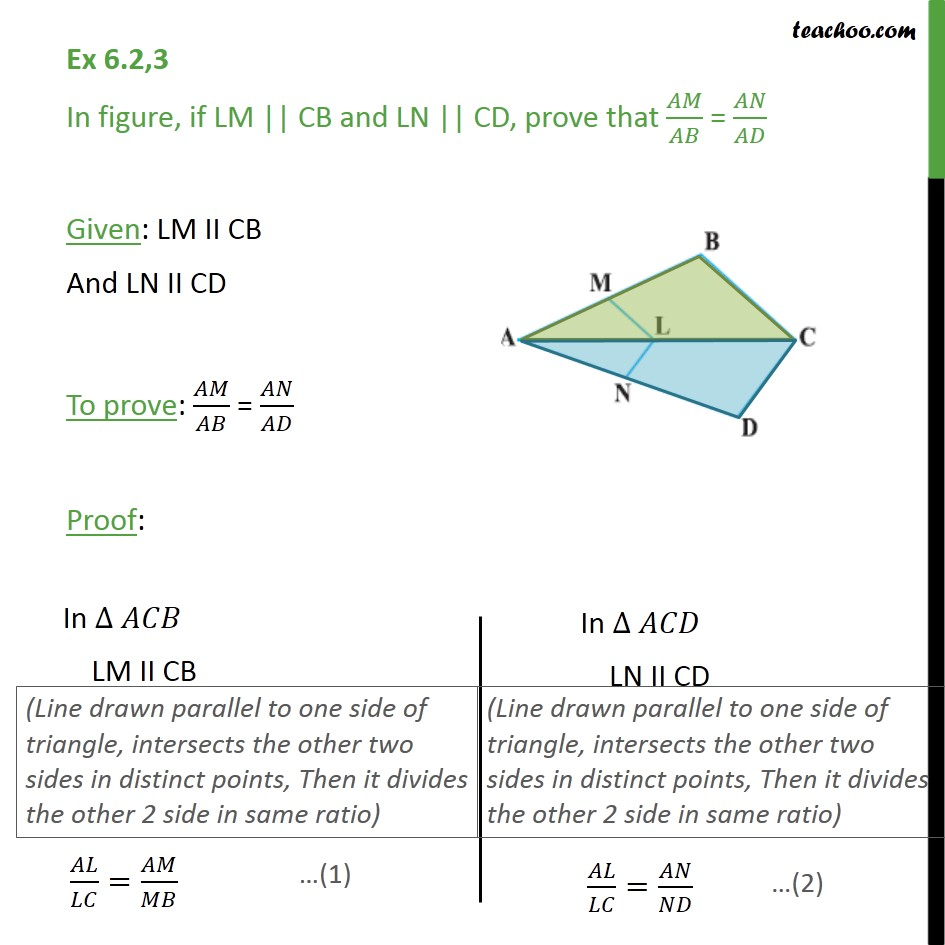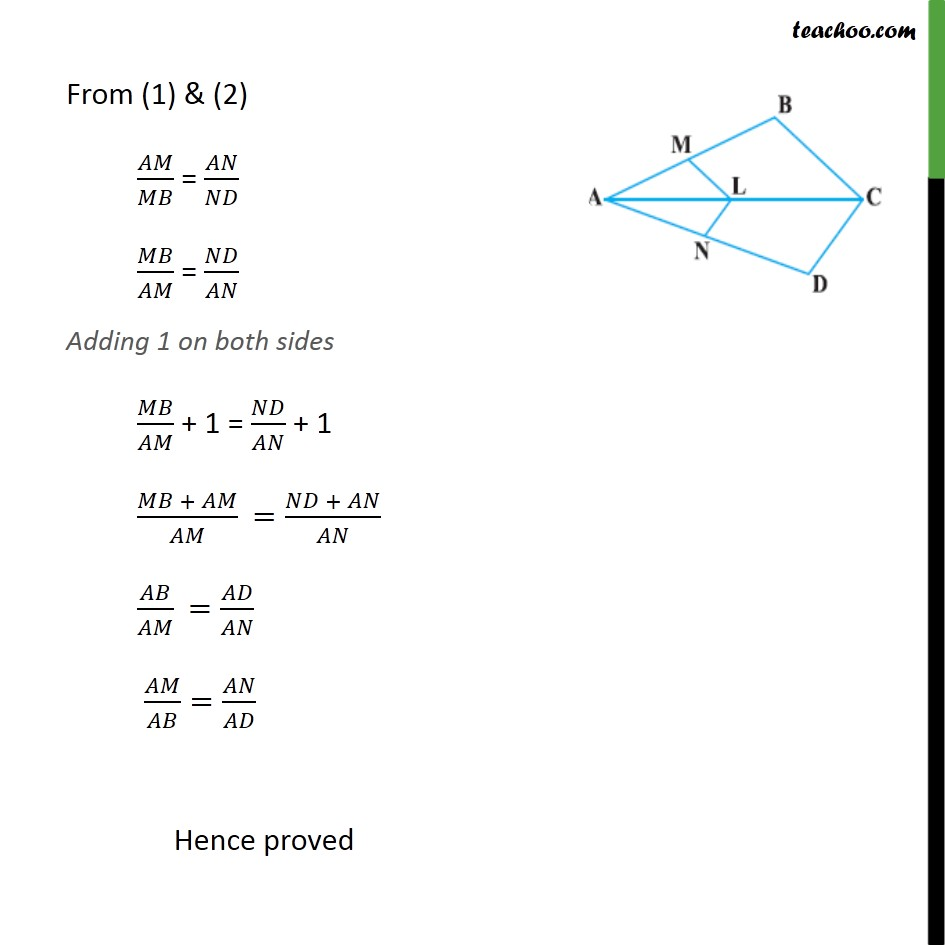1. Chapter 6 Class 10 Triangles (Term 1)
2. Serial order wise
3. Ex 6.2

Transcript

Ex 6.2,3 In figure, if LM || CB and LN || CD, prove that 𝐴𝑀/𝐴𝐵 = 𝐴𝑁/𝐴𝐷 Given: LM II CB And LN II CD To prove: 𝐴𝑀/𝐴𝐵 = 𝐴𝑁/𝐴𝐷 Proof: From (1) & (2) 𝐴𝑀/𝑀𝐵 = 𝐴𝑁/𝑁𝐷 𝑀𝐵/𝐴𝑀 = 𝑁𝐷/𝐴𝑁 Adding 1 on both sides 𝑀𝐵/𝐴𝑀 + 1 = 𝑁𝐷/𝐴𝑁 + 1 (𝑀𝐵 + 𝐴𝑀)/𝐴𝑀 =(𝑁𝐷 + 𝐴𝑁)/𝐴𝑁 𝐴𝐵/𝐴𝑀 =𝐴𝐷/𝐴𝑁 𝐴𝑀/𝐴𝐵=𝐴𝑁/𝐴𝐷 Hence proved